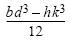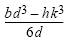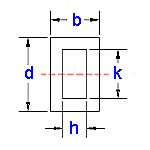### Area Moment of Inertia Section Properties: Rectangle Tube Calculator

Area Moment of Inertia Section Properties: Rectangle Tube Calculator

Area Moment of Inertia Section Properties of Rectangle Tube Calculator Calculator and Equations. This engineering calculator will determine the section modulus for the given cross-section. This engineering data is often used in the design of structural beams or structural flexural members.

 Description Equation Area Moment of Inertia Section Properties = ISection Modulus = Z = I/yRadius of GyrationA = area A = db - hk y = Distance to neutral axis y = d/2Area Moment of Inertia Section Properties: Rectangle Tube Variables Inputs: Inch (in.) Metric (mm) "b" (Width) = "d" (Height) = "h" (Width) = "k" (Height) = Properties: Area Moment of Inertia Section Properties (Units4) = Section Modulus (Units3) = Radius of Gyration (Units) = Extreme Point (Units) = Area (Units2) =